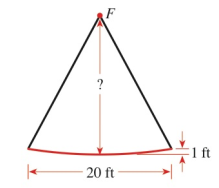Chapter 12.1, Problem 62E### Algebra and Trigonometry (MindTap ...

4th Edition
James Stewart + 2 others
ISBN: 9781305071742

#### Solutions

Chapter
Section### Algebra and Trigonometry (MindTap ...

4th Edition
James Stewart + 2 others
ISBN: 9781305071742
Textbook Problem

# APPLICATIONSSatellite Dish A reflector for a satellite dish is parabolic in cross section, with the receiverat the focus F . The reflector is 1   ft deep and 20   ft wide from rim to rim (see the figure). How far is the receiver from the vertex of the parabolic reflector?To determine

To Find:

The distance between the receiver and vertex of parabolic reflector.

Explanation

Given:

A reflector for a satellite dish is parabolic in cross section, with the receiver at the focus F is shown in figure (1).

Figure (1)

The depth of reflector is 1 ft. and has width of 20 ft. from rim to rim.

Concept:

The general equation of a parabola with vertical axis where vertex V(0,0) and focus at F(0,p) is given by,

x2=4py …… (1)

Where y=p is the directrix.

1) If p<0, then parabola opens downward.

2)If p>0, then parabola opens upward.

Width of a parabola is given by |2x|.

Calculation:

Since, given parabola is drawn with vertical axis, the general equation will be given as,

x2=4py

As the parabola opens upward, p>0

### Still sussing out bartleby?

Check out a sample textbook solution.

See a sample solution

#### The Solution to Your Study Problems

Bartleby provides explanations to thousands of textbook problems written by our experts, many with advanced degrees!

Get Started